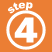Home    |    Teacher    |    Parents    |    Glossary    |    About UsAre these statements true or false? True False Feedback "Pi" is a real number. 2.52 is a real, rational number. n + 5 is an expression for the sum of a number and five. 3 is an irrational number. Twice a number divided by three can be written as 2n - 3. Five decreased by twice a number can be written as 5 - 2x. Ten less than a number can be written as 10 n. An integer is not a rational number.
 Homework Help | Algebra | The Language of AlgebraEmail this page to a friendSearch·  Definitions·  Order of Operations·  Writing equations·  Writing inequalitiesFirst Glance In Depth Examples WorkoutDefinitions